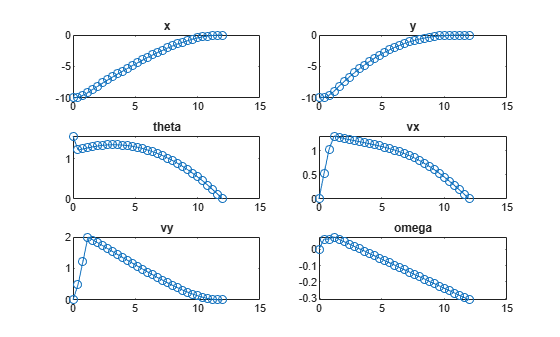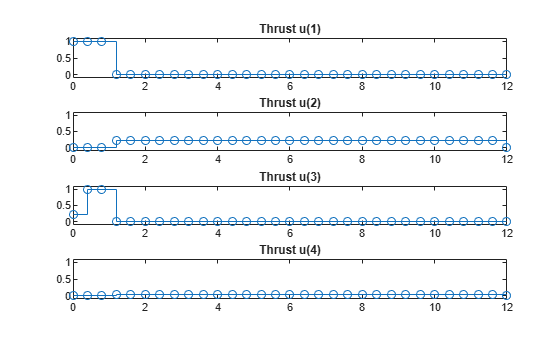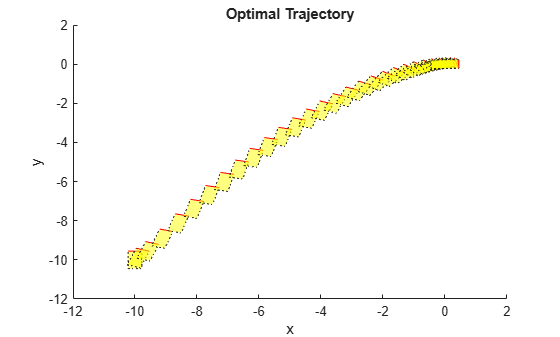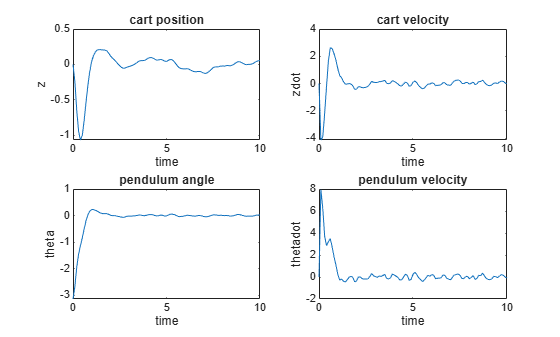Documentation

# nlmpcmove

Compute optimal control action for nonlinear MPC controller

## Syntax

``mv = nlmpcmove(nlmpcobj,x,lastmv)``
``mv = nlmpcmove(nlmpcobj,x,lastmv,ref)``
``mv = nlmpcmove(nlmpcobj,x,lastmv,ref,md)``
``mv = nlmpcmove(nlmpcobj,x,lastmv,ref,md,options)``
``[mv,opt] = nlmpcmove(___)``
``[mv,opt,info] = nlmpcmove(___)``

## Description

````mv = nlmpcmove(nlmpcobj,x,lastmv)` computes the optimal manipulated variable control action for the current time. To simulate closed-loop nonlinear MPC control, call `nlmpcmove` repeatedly.```

example

````mv = nlmpcmove(nlmpcobj,x,lastmv,ref)` specifies reference values for the plant outputs. If you do not specify reference values, `nlmpcmove` uses zeros by default.```
````mv = nlmpcmove(nlmpcobj,x,lastmv,ref,md)` specifies run-time measured disturbance values. If your controller has measured disturbances, you must specify `md`.```

example

````mv = nlmpcmove(nlmpcobj,x,lastmv,ref,md,options)` specifies additional run-time options for computing optimal control moves. Using `options`, you can specify initial guesses for state and manipulated variable trajectories, update tuning weights at constraints, or modify prediction model parameters.```

example

````[mv,opt] = nlmpcmove(___)` returns an `nlmpcmoveopt` object that contains initial guesses for the state and manipulated trajectories to be used in the next control interval.```

example

````[mv,opt,info] = nlmpcmove(___)` returns additional solution details, including the final optimization cost function value and the optimal manipulated variable, state, and output trajectories.```

## Examples

collapse all

Create nonlinear MPC controller with six states, six outputs, and four inputs.

```nx = 6; ny = 6; nu = 4; nlobj = nlmpc(nx,ny,nu);```
```In standard cost function, zero weights are applied by default to one or more OVs because there are fewer MVs than OVs. ```

Specify the controller sample time and horizons.

```Ts = 0.4; p = 30; c = 4; nlobj.Ts = Ts; nlobj.PredictionHorizon = p; nlobj.ControlHorizon = c;```

Specify the prediction model state function and the Jacobian of the state function. For this example, use a model of a flying robot.

```nlobj.Model.StateFcn = "FlyingRobotStateFcn"; nlobj.Jacobian.StateFcn = "FlyingRobotStateJacobianFcn";```

Specify a custom cost function for the controller that replaces the standard cost function.

```nlobj.Optimization.CustomCostFcn = @(X,U,e,data) Ts*sum(sum(U(1:p,:))); nlobj.Optimization.ReplaceStandardCost = true;```

Specify a custom constraint function for the controller.

`nlobj.Optimization.CustomEqConFcn = @(X,U,data) X(end,:)';`

Specify linear constraints on the manipulated variables.

```for ct = 1:nu nlobj.MV(ct).Min = 0; nlobj.MV(ct).Max = 1; end```

Validate the prediction model and custom functions at the initial states (`x0`) and initial inputs (`u0`) of the robot.

```x0 = [-10;-10;pi/2;0;0;0]; u0 = zeros(nu,1); validateFcns(nlobj,x0,u0);```
```Model.StateFcn is OK. Jacobian.StateFcn is OK. No output function specified. Assuming "y = x" in the prediction model. Optimization.CustomCostFcn is OK. Optimization.CustomEqConFcn is OK. Analysis of user-provided model, cost, and constraint functions complete. ```

Compute the optimal state and manipulated variable trajectories, which are returned in the `info`.

`[~,~,info] = nlmpcmove(nlobj,x0,u0);`
```Slack variable unused or zero-weighted in your custom cost function. All constraints will be hard. ```

Plot the optimal trajectories.

`FlyingRobotPlotPlanning(info)`
```Optimal fuel consumption = 4.712383 ```Create a nonlinear MPC controller with four states, two outputs, and one input.

`nlobj = nlmpc(4,2,1);`
```In standard cost function, zero weights are applied by default to one or more OVs because there are fewer MVs than OVs. ```

Specify the sample time and horizons of the controller.

```Ts = 0.1; nlobj.Ts = Ts; nlobj.PredictionHorizon = 10; nlobj.ControlHorizon = 5;```

Specify the state function for the controller, which is in the file `pendulumDT0.m`. This discrete-time model integrates the continuous time model defined in `pendulumCT0.m` using a multistep forward Euler method.

```nlobj.Model.StateFcn = "pendulumDT0"; nlobj.Model.IsContinuousTime = false;```

The prediction model uses an optional parameter, `Ts`, to represent the sample time. Specify the number of parameters.

`nlobj.Model.NumberOfParameters = 1;`

Specify the output function of the model, passing the sample time parameter as an input argument.

`nlobj.Model.OutputFcn = @(x,u,Ts) [x(1); x(3)];`

Define standard constraints for the controller.

```nlobj.Weights.OutputVariables = [3 3]; nlobj.Weights.ManipulatedVariablesRate = 0.1; nlobj.OV(1).Min = -10; nlobj.OV(1).Max = 10; nlobj.MV.Min = -100; nlobj.MV.Max = 100;```

Validate the prediction model functions.

```x0 = [0.1;0.2;-pi/2;0.3]; u0 = 0.4; validateFcns(nlobj, x0, u0, [], {Ts});```
```Model.StateFcn is OK. Model.OutputFcn is OK. Analysis of user-provided model, cost, and constraint functions complete. ```

Only two of the plant states are measurable. Therefore, create an extended Kalman filter for estimating the four plant states. Its state transition function is defined in `pendulumStateFcn.m` and its measurement function is defined in `pendulumMeasurementFcn.m`.

`EKF = extendedKalmanFilter(@pendulumStateFcn,@pendulumMeasurementFcn);`

Define initial conditions for the simulation, initialize the extended Kalman filter state, and specify a zero initial manipulated variable value.

```x = [0;0;-pi;0]; y = [x(1);x(3)]; EKF.State = x; mv = 0;```

Specify the output reference value.

`yref = [0 0];`

Create an `nlmpcmoveopt` object, and specify the sample time parameter.

```nloptions = nlmpcmoveopt; nloptions.Parameters = {Ts};```

Run the simulation for `10` seconds. During each control interval:

1. Correct the previous prediction using the current measurement.

2. Compute optimal control moves using `nlmpcmoveopt`. This function returns the computed optimal sequences in `nloptions`. Passing the updated options object to `nlmpcmoveopt` in the next control interval provides initial guesses for the optimal sequences.

3. Predict the model states.

4. Apply the first computed optimal control move to the plant, updating the plant states.

5. Generate sensor data with white noise.

6. Save the plant states.

```Duration = 10; xHistory = x; for ct = 1:(Duration/Ts) % Correct previous prediction xk = correct(EKF,y); % Compute optimal control moves [mv,nloptions] = nlmpcmove(nlobj,xk,mv,yref,[],nloptions); % Predict prediction model states for the next iteration predict(EKF,[mv; Ts]); % Implement first optimal control move x = pendulumDT0(x,mv,Ts); % Generate sensor data y = x([1 3]) + randn(2,1)*0.01; % Save plant states xHistory = [xHistory x]; end```

Plot the resulting state trajectories.

```figure subplot(2,2,1) plot(0:Ts:Duration,xHistory(1,:)) xlabel('time') ylabel('z') title('cart position') subplot(2,2,2) plot(0:Ts:Duration,xHistory(2,:)) xlabel('time') ylabel('zdot') title('cart velocity') subplot(2,2,3) plot(0:Ts:Duration,xHistory(3,:)) xlabel('time') ylabel('theta') title('pendulum angle') subplot(2,2,4) plot(0:Ts:Duration,xHistory(4,:)) xlabel('time') ylabel('thetadot') title('pendulum velocity')```## Input Arguments

collapse all

Nonlinear MPC controller, specified as an `nlmpc` object.

Current prediction model states, specified as a vector of lengthNx, where Nx is the number of prediction model states. Since the nonlinear MPC controller does not perform state estimation, you must either measure or estimate the current prediction model states at each control interval.

Control signals used in plant at previous control interval, specified as a vector of lengthNmv, where Nmv is the number of manipulated variables.

### Note

Specify `last_mv` as the MV signals applied to the plant in the previous control interval. Typically, these MV signals are the values generated by the controller, though this is not always the case. For example, if your controller is offline and running in tracking mode; that is, the controller output is not driving the plant, then feeding the actual control signal to `last_mv` can help achieve bumpless transfer when the controller is switched back online.

Plant output reference values, specified as a row vector of length Ny or an array with Ny columns, where Ny is the number of output variables. If you do not specify `ref`, the default reference values are zero.

To use the same reference values across the prediction horizon, specify a row vector.

To vary the reference values over the prediction horizon from time k+1 to time k+p, specify an array with up to p rows. Here, k is the current time and p is the prediction horizon. Each row contains the reference values for one prediction horizon step. If you specify fewer than p rows, the values in the final row are used for the remaining steps of the prediction horizon.

Measured disturbance values, specified as a row vector of length Nmd or an array with Nmd columns, where Nmd is the number of measured disturbances. If your controller has measured disturbances, you must specify `md`. If your controller has no measured disturbances, specify `md` as `[]`.

To use the same disturbance values across the prediction horizon, specify a row vector.

To vary the disturbance values over the prediction horizon from time k to time k+p, specify an array with up to p+1 rows. Here, k is the current time and p is the prediction horizon. Each row contains the disturbance values for one prediction horizon step. If you specify fewer than p rows, the values in the final row are used for the remaining steps of the prediction horizon.

Run-time options, specified as an `nlmpcmoveopt` object. Using these options, you can:

• Tune controller weights

• Update linear constraints

• Set manipulated variable targets

• Specify prediction model parameters

• Provide initial guesses for state and manipulated variable trajectories

These options apply to only the current `nlmpcmove` time instant.

To improve solver efficiency, it is best practice to specify initial guesses for the state and manipulated variable trajectories.

## Output Arguments

collapse all

Optimal manipulated variable control action, returned as a column vector of length Nmv, where Nmv is the number of manipulated variables.

If the solver converges to a local optimum solution (`info.ExitFlag` is positive), then `mv` contains the optimal solution.

If the solver reaches the maximum number of iterations without finding an optimal solution (`info.ExitFlag = 0`) and:

• `nlmpcobj.Optimization.UseSuboptimalSolution` is `true`, then `mv` contains the suboptimal solution

• `nlmpcobj.Optimization.UseSuboptimalSolution` is `false`, then `mv` contains `lastmv`

If the solver fails (`info.ExitFlag` is negative), then `mv` contains `lastmv`.

Run-time options with initial guesses for the state and manipulated variable trajectories to be used in the next control interval, returned as an `nlmpcmoveopt` object. Any run-time options that you specified using `options`, such as weights, constraints, or parameters, are copied to `opt`.

The initial guesses for the states (`opt.X0`) and manipulated variables (`opt.MV0`) are the optimal trajectories computed by `nlmpcmove` and correspond to the last p-1 rows of `info.Xopt` and `info.MVopt`, respectively.

To use these initial guesses in the next control interval, specify `opt` as the `options` input argument to `nlmpcmove`.

Solution details, returned as a structure with the following fields.

Optimal manipulated variable sequence, returned as a (p+1)-by-Nmv array, where p is the prediction horizon and Nmv is the number of manipulated variables.

`MVopt(i,:)` contains the calculated optimal manipulated variable values at time `k+i-1`, for ```i = 1,...,p```, where `k` is the current time. `MVopt(1,:)` contains the same manipulated variable values as output argument `mv`. Since the controller does not calculate optimal control moves at time `k+p`, `MVopt(p+1,:)` is equal to `MVopt(p,:)`.

Optimal prediction model state sequence, returned as a (p+1)-by-Nx array, where p is the prediction horizon and Nx is the number of states in the prediction model.

`Xopt(i,:)` contains the calculated state values at time `k+i-1`, for `i = 2,...,p+1`, where `k` is the current time. `Xopt(1,:)` is the same as the current states in `x`.

Optimal output variable sequence, returned as a (p+1)-by-Ny array, where p is the prediction horizon and Ny is the number of outputs.

`Yopt(i,:)` contains the calculated output values at time `k+i-1`, for `i = 2,...,p+1`, where `k` is the current time. `Yopt(1,:)` is computed based on the current states in `x` and the current measured disturbances in `md`, if any.

Prediction horizon time sequence, returned as a column vector of length p+1, p is the prediction horizon. `Topt` contains the time sequence from time k to time k+p, where k is the current time.

`Topt(1)` = 0 represents the current time. Subsequent time steps `Topt(i)` are `Ts*(i-1)`, where `Ts = nlmpcobj.Ts` is the controller sample time.

Use `Topt` when plotting `Uopt`, `Xopt`, or `Yopt` sequences.

Slack variable, ε, used in constraint softening, returned as a nonnegative scalar value.

• ε = 0 — All soft constraints are satisfied over the entire prediction horizon.

• ε > 0 — At least one soft constraint is violated. When more than one constraint is violated, ε represents the worst-case soft constraint violation (scaled by your ECR values for each constraint).

Optimization exit code, returned as one of the following:

• Positive Integer — Solver converged to an optimal solution

• `0` — Maximum number of iterations reached without converging to an optimal solution

• Negative integer — Solver failed

Objective function cost, returned as a nonnegative scalar value. The cost quantifies the degree to which the controller has achieved its objectives.

The cost value is only meaningful when `ExitFlag` is nonnegative.

## Tips

During closed-loop simulations, it is best practice to warm start the nonlinear solver by using the predicted state and manipulated variable trajectories from the previous control interval as the initial guesses for the current control interval. To use these trajectories as initial guesses:

1. Return the `opt` output argument when calling `nlmpcmove`. This `nlmpcmoveopt` object contains any run-time options you specified in the previous call to `nlmpcmove`, along with the initial guesses for the state (`opt.X0`) and manipulated variable (`opt.MV0`) trajectories.

2. Pass this object in as the `options` input argument to `nlmpcmove` for the next control interval.

These steps are a best practice, even if you do not specify any other run-time options.

## See Also

### Topics

#### Implementing an Adaptive Cruise Controller with Simulink

Download technical paper